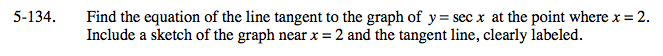### Home > CALC > Chapter 5 > Lesson 5.3.3 > Problem5-134

5-134.

Find the equation of the line tangent to the graph of y = sec x at the point where x = 2. Include a sketch of the graph near x = 2 and the tangent line, clearly labeled. Homework Help ✎Use point-slope form of a line.

The original equation gives you the point of tangency. The derivative gives you the slope.